﻿ (Lantool) Power Generation Cost Minimization Software Application

### (Lantool) Power Generation Cost Minimization Software Application

Anireh V.I.E., John Tarilanyo AfaOPEN ACCESSPEER-REVIEWED

## (Lantool) Power Generation Cost Minimization Software Application

Anireh V.I.E.1,, John Tarilanyo Afa2

1Department of Electrical/Computer Engineering, Rivers State University of Science and Technology, Rivers State, Nigeria

2Department of Electrical/Electronic Engineering, Niger Delta University, Bayelsa State, Nigeria

### Abstract

Managers of large scale industry like the electricity generation are challenged at many fronts because the tasks involved are complex, influenced by unexpected events and evolve in time. Currently the application of computer methods in this industry has produced tremendous positive results. In this regard, the study presents an effort developed to solve optimal power flow (economic dispatch) problem by minimizing the cost of generation using the lagrangian multiplier method. A decision support system ‘Lantool’ is presented. The approach is validated by lagrangian method found in technical literatures. The system will assist operators in thermal power plants with the task of planning generation in the most economic way. A result obtained from the application explains the important role decision support systems can play in the management of the electricity generation industry.

### At a glance: Figures

12
Prev Next

• V.I.E., Anireh, and John Tarilanyo Afa. "(Lantool) Power Generation Cost Minimization Software Application." American Journal of Electrical and Electronic Engineering 2.1 (2014): 21-26.
• V.I.E., A. , & Afa, J. T. (2014). (Lantool) Power Generation Cost Minimization Software Application. American Journal of Electrical and Electronic Engineering, 2(1), 21-26.
• V.I.E., Anireh, and John Tarilanyo Afa. "(Lantool) Power Generation Cost Minimization Software Application." American Journal of Electrical and Electronic Engineering 2, no. 1 (2014): 21-26.

 Import into BibTeX Import into EndNote Import into RefMan Import into RefWorks

### 1. Introduction

The rapid growth in power system industry has made the issue of optimization in power generation and transmission very significant. In the recent time, deregulation in power industry has changed the part played by various units in the electricity industry. Services provided by electric power vendors which originally used to be one single entity are now unbundled into three different entities thus production, transmission and distribution. The service providers’ compete in providing good electricity delivery and at the same time strive to make profit. To maintain efficiency and security, reliability of supply are not compromised otherwise customer interest and protection will not be guaranteed. In other to achieve these, best practices in economic dispatch  or optimal power flow are employed. The objective is to systematically seek the lowest cost of electricity production that will be consistent with electricity demand. To minimize cost, Optimal power flow (OPF) will increase the use of more efficient generating unit and at the same time addresses two issues of concern - better fuel usage, and reduced green house gas emission [2, 3, 4] that would result from less efficient generation. OPF therefore seeks to minimize the total cost of generating power (production cost) at various stations while satisfying the loads and the losses in the transmission lines. In the case of developing countries like Nigeria where consumption per capita of electricity is very poor mainly due to high cost of production , application of the principles of OPF cannot be over looked. Even when these principles are to be employed the approach to deliver it in a simpler and user friendly manner to enable operators effectively apply it is equally very necessary. It is in view of all these considerations that this paper seeks to present a computer based support system for the electricity generation industry. Computer applications in the form decision support system (DSS) can be a tool used to provide fair and consistent decisions, and at the same time improve the effectiveness of decision making process . A DSS application approach for supporting decision making is typically built for solutions of a certain problem or to evaluate an opportunity. On that note, we present a decision support computer-based tool using Lagrangian multiplier method. The approach was applied to a five-bus network to minimize cost of electricity generation and result is also presented.

Many solution techniques have been proposed and also available to solving optimal power flow problem with varied degree of successes. They can be divided into two main categories, the algorithmic mathematical solution and artificial intelligent solution as reported in several literatures. Among the algorithmic solutions are Interior point (IP) algorithm , Simplex algorithm (SA), Quadratic programming (QP), and Dynamic programming (DP) . Langrange relaxation method (LRM) [9, 10] Linear programming (LP), Non-linear programming (NLP) and Newton-based methods have also been reported. Currently proposed Artificial Intelligent optimization methods based on heuristics and operational research presented by researchers have emerged with global optimum solution for power system optimization. They include Expert system (ES), Ant Colony search (ACS) , Simulated annealing (SA) , Artificial Neural networks (ANN) [13, 14, 15], Fuzzy logic (FL)  and Genetic Algorithm (GA) . Others include Meta heuristic methods such as Tabu search (TS) , Particle swarm optimization (PSO) [19, 20] and Evolutionary programming (EP) [21, 22]. Application of these methods depends on the researchers’ area of interest as each method has its own advantages and disadvantages. Even as these optimization methods are effective in finding the global optimal solution, however, when adopted in large-scale real-world system, it would take a long computational time. It therefore explains the fact that there exist a gap between theory and practice in the field of optimization. Attempt to bridge this gap is what this paper seek to present by shifting focus to applying analytical method to solving a real-world problem.

1.1. OPF Formulations

Generally, power system can be operated optimally if the principles of equal incremental cost rate are applied to the problem of optimal distribution of loads among the various stations forming a power pool. The most precise way to describe such operation is to use the relation between the input and output parameter as available from the generated data of the individual generators.

The characteristic of this relation is described mathematically as.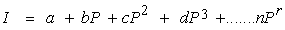(1)

Where I is the input (cost of fuel) and P is the output (power produced by generator) with positive coefficients a, b, c to n.

Alternatively it can be represented graphically as shown in Figure 1:

I0 in the above graph represents the amount of input required to keep the generator functioning when there is no load. The slopes of the curve at various load-points give the incremental cost rate. If I1 and I2 are the inputs corresponding to the loads P1 and P2 respectively, the increase in input required for meeting the increase of load from P1 to P2 is given by(2)

The area under the curve between P1 and P2 is the incremental rate. Similarly, from no load to P1, the increase in input I1 – I0 is given by the area under the incremental rate curve from P = 0 to P1, and Ri is the incremental rate.

By differentiating the expression in equation 1 to the third power, we get the incremental rate characteristic as:(3)

However, the theory of incremental rate assumes the following conditions:

a. That input – output curves are continuous

b. That first derivatives of the input – output curves are continuous

c. That value of the incremental rate increases with the increase in output

Hence to minimize I (input) the condition will be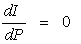for generators i = 1 --------- n.

Then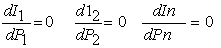(4)

Hence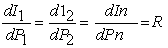(5)

Therefore the input will be a minimum for a specified load when the units have the same incremental rate.

### 2. Solution to Minimizing Cost of Generation

With the above background information the objective of generation cost minimization is to schedule generation such that input (I) is minimum for the given total power P, subject to restriction that the sum of Pk = P (is the total load received), where Pk is the output of unit k.

Using the lagrangian method, if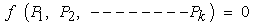(6)

And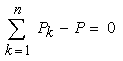(7)

Then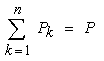If I represent the cost of input, the minimum input cost is realized when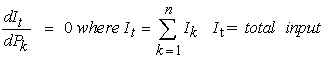Applying lagrangian type multiplier where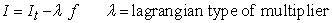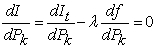(8)

Where f is the function of total power received ie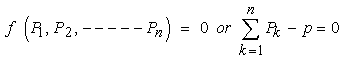But RHS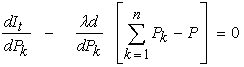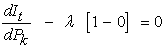(9)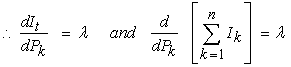Hence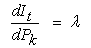(10)

The incremental cost of input to kth unit in dollar per hour is equal to the incremental cost of the received power.

The equation 10 may be rewritten as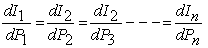(11)

If the incremental rate of kth unit is written as Rik, then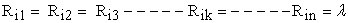(12)

Using symbol, C, for incremental production cost in dollar per hour then: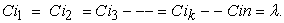(13)

Where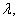the lagrangian multiplier, is the incremental cost of received power in dollar per hour.

In other words, suppose we have a five-bus power system network in a one line diagram as shown in Figure 2, we can develop an computer based algorithm for the optimal distribution cost using the above an analytical solution.

2.1. Algorithm for the Optimal Power Flow of the Five-Bus Network

For x MW to be generated from PG1, PG2, and PG3 (where the PGis are of equal capacity) that would service the combined external load y in PD3, PD4 optimally i.e. x = y, we proceed follows:

1. Obtain the cost functions for each of the generators PG1, PG2, PG3 as follows: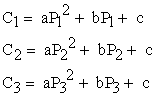2. for optimal economic allocation of load y we apply the lagrangian method, where: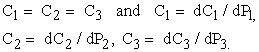3. differentiate the C1, C2, and C3 with respect to PG1, PG2, and PG3.

4. solve for P1, P2, and P3 by equating the differential for minimum cost.

5. add P1, P2, and P3 the total will be equal to y, the total load to service i.e P1+ P2+P3 = y.

2.2. Algorithm for the Optimal Cost Savings in the Five-Bus Network

To obtain cost saving effected by the optimal allocation instead of equal division of load we proceed as:

1. let PG1', PG2' and PG3' = x' (x' is one-third of x and (PGi')’s are of equal capacity).

2. obtain the cost function (Ci')’s of P1' P2' P3' as follows: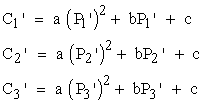3. obtain increased cost of input for P1' as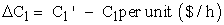4. Saving in cost of input for P2'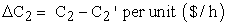5. Saving in cost of input for P3'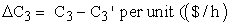6. Net increase cost is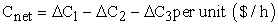7. for 24 hours we obtain Cday = Cnet * 24 hours, Cday is the daily cost saving for running the three generators.

### 3. Results

Results obtained from ‘Lantool’ decision support application is shown in Table 2 for various load demands. As expected the difference between operating the station optimally as against equal distribution is clearly identified in the table as NetSave. The daily cost saving (NetSave) for each of the load demands per day is also shown.

### 4. Discussion

In the analysis of optimal load division between the various units of a plant, it is expected that the available data on cost is absolutely correct. The implementation of the ‘Lantool’ program is based on data set for the test cases of Table 1. The table shows generator input cost data as C1, C2, C3, while P1, P2, P3, are power generated data for Pg1, Pg2, and Pg3 respectively for the five-bus network in Figure 2. The input cost function is derived from equation 3 with all coefficients positive. (The coefficients for input cost equations are obtained using Matlab Curve Fitting Toolbox). Line plots of cost versus power output is shown in Figure 3. The optimum economy is achieved if every unit (i.e. Pg1, Pg2, and Pg3) operates at the same incremental cost(IC) as seen in Figure 4. At any point on the incremental cost plot, the three generators are operated optimally and fuel utilization is seen to be less. Screen shots of cost with output of simulation, daily cost saving and incremental cost plot/data is shown in Figure 5 and Figure 6. The output of daily net saving per load demand when multiplied by 30 days will give the cost savings per month.

#### Table 2. Sample result of Optimal vs Equal distribution cost from LanTool per load demand

Figure 5. Sample screen for the lagrangian Optimal/Equal distribution cost with output of simulation and daily cost saving data
Figure 6. Sample screen for the lagrangian Optimal/Equaldistribution cost with output of simulation, daily cost saving and incremental cost plot/data

### 5. Conclusion

Minimizing cost of fuel in generating electricity is a real world problem and requires practical solution. The paper presented lantool, a decision support application tool designed to determine the best combination of power generating plant to produce electricity with less fuel cost. It was formulated using the lagrangian multiplier method and developed as an interactive application for operators at gas turbine generating (GTG) plant. The design uses a special object oriented methodology feature of MatLab called handle graphics technology (HGT). This approach provides the application with menu-driven guides that would enable operators with little knowledge of computer navigate through it without difficulty. Lantool can be extended to include an embedded form. When interfaced with a sensor can automatically control the switching of power plant for optimal operation. Simulation data from other stations tested on lantool show no significant difference in result implying that the system can be deployed in a dissimilar geographical location.

### References

  Dongxiao N, Yanan W. “A novel social-environmental-economic dispatch model for thermal/wind power generation and application” International Journal of Innovative Computing, Information and Control (ICIC). 9(7), 2013.In article  Alrashidi M.A, Hawary M.E., “Impact of loading conditions on the emission economic dispatch”. Proceedings of World Academy of Science, Engineering and Technology, 2008.In article  Wang L, Chanan S., “Reserve constrained multiarea environmental/economic dispatch based on particle swarm optimization with local search”. Engineering Application of Artificial Intelligence Science Direct, 2008.In article  Peixoto, H.M., Guerreiro, A.M.G., Dória Neto, A.D., “Image processing for eye detection and classification of the gaze direction”, Proceedings of the 2009 International Joint Conference on Neural Networks, Atlanta, GA, USA. pp. 2475-2480, 2009.In article CrossRef  Sambo A.S., “The Challenges of Sustainable Energy Development in Nigeria”. Presentation to Nigerian Society of Engineers, Abuja, Nigeria, 2009.In article  Palma-dos-Reis, A., Zahedi, F. M., “Designing personalized intelligent financial support systems”. Decision Support System, 26(1): 31-47, 1999.In article CrossRef  Martinez L.J., Lora T.A., Santos R.J., “Short term hydrothermal coordination based on interior point nonlinear programing andgenetic Algorithm”. IEEE Porto Power Tech Conference, 2001.In article  Shi C.C., Chun H.C., Fomg I-K, Lah P.B., “Hydroelectric generation scheduling with an effective differential dynamic programming algorithm”. IEEE Transactions on Power System, 1990.In article  Erion F., Silva E, Laudia S., “Solving the unit commitment problem of hydropower plants via Lagrangian relaxation and sequential quadratic programming”. Journal of Computational & Applied Mathematics, 2005.In article  Tkayuki S., Kamu, W., Lagrangian relaxation method for price based unit commitment Problem. Engineering Optimization, Taylor Francis, 2004.In article  Huang J.S., “Enhancement of hydroelectric Generation scheduling using ant colony system based optimization approaches”. IEEE Transactions on Energy Conversion, 2001.In article  Mantawy A, Abdel-Magid Y., Selim S., “A simulated annealing algorithm for unit commitment”, IEEE Trans. Power Syst., 13(1): 197-204, 1998.In article CrossRef  Naresh R, Sharma J., “Short term hydro scheduling using two phase neural network”. International Journal of Electrical Power and Energy Systems, 2002.In article CrossRef  Liu, D., Dong X., Shi, P., “The optimization based on improved membrane algorithm for fuzzy RBF neural network control of ball-plate system”, ICIC Express Letters, Part B: Applications, 3(5): 1259-1267, 2012.In article  Lopes, D.C., “Implementation of a Modular Neural Network in a Multiple Processor System on FPGA to Classify Electric Disturbance”. Industrial Electronics. IECON '09. 35th Annual Conference of IEEE, Porto, Portugal, 2009.In article  Lee, T.E, Su, J.P., Yu K.W., “Fuzzy gain scheduled alpha-beta-gamma filter design based on particle swarm optimization method”, ICIC Express Letters, 4(6B): 2305-2310, 2010.In article  Kazarlis, S., Bakirtzis, A., Petridis, V., “A genetic algorithm solution to the unit commitment problem”, IEEE Trans. Power Syst., 11(1): 83-92, 1996.In article CrossRef  Lin, W.M, Cheng, F.S, Tsay, M.T., “An improved Tabu search for economic dispatch with multiple minima”. IEEE Transaction on Power System, 2002.In article  Jie, X, Deyun, X., “New Metropolis coefficients of particle swarm optimization”, IEEE, 2008.In article  Liao, G., “A novel evolutionary algorithm for dynamic economic dispatch with energy saving and emission reduction in power system integrated wind power”, Energy, 36: 1018-1029, 2011.In article CrossRef  Yang, H.T, Yang, P.C, Huang, C. L., “Evolutionary programming based economic dispatch for units with non smooth fuel cost function”, IEEE Transaction on Power System, 1996.In article  Information and Telecommunication Technologies Peixoto, H.M., Cruz, R. M.S., Guerreiro, A.M.G., Dória Neto, A.D., D’Assunção, A.G., “A comparison of multilayer perceptrons training algorithms for the optimization of frequency selective surfaces”, Proceedings of 8th International Symposium, Florianopolis, SC, Brazil, 2009.In article  Gangadhar, K.A. “Electrical Power Systems”. Khanna Publishers. Nai,Sarak, Delhi. India, 2006.In article